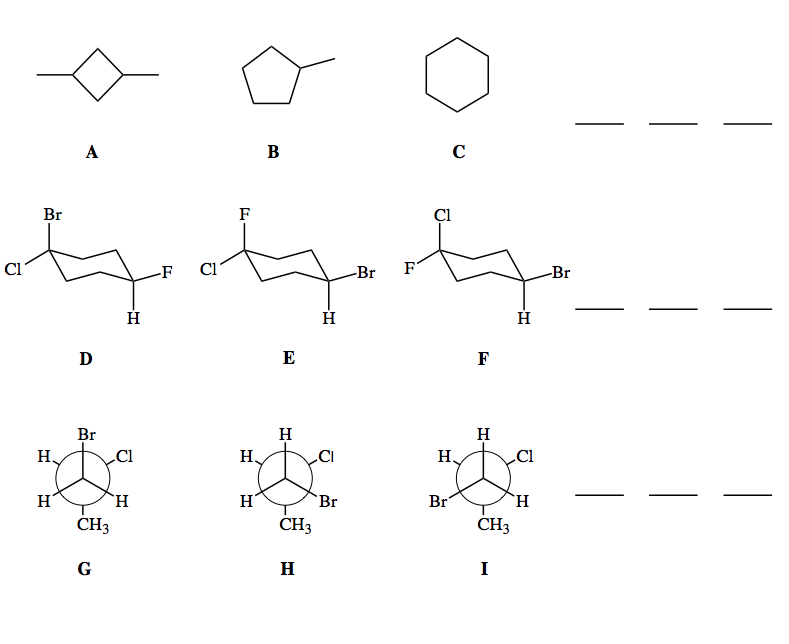# Problem: Put each threesome below in order of stability from high to low using &gt; or = signs. If two species are equal use = signs.

###### FREE Expert Solution
83% (295 ratings)
###### Problem Details

Put each threesome below in order of stability from high to low using > or = signs. If two species are equal use = signs.Frequently Asked Questions

What scientific concept do you need to know in order to solve this problem?

Our tutors have indicated that to solve this problem you will need to apply the Equatorial Preference concept. You can view video lessons to learn Equatorial Preference. Or if you need more Equatorial Preference practice, you can also practice Equatorial Preference practice problems.

What is the difficulty of this problem?

Our tutors rated the difficulty ofPut each threesome below in order of stability from high to ...as medium difficulty.

What professor is this problem relevant for?

Based on our data, we think this problem is relevant for Professor Wenthold's class at PURDUE.